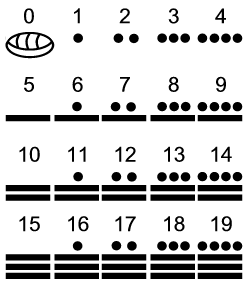## MTH211 Foundations of Elementary Math 1### Fun with whole numbers.

Notes on Whole Numbers
Fall 2019

### Chapter 3: Whole Numbers

The Common Core State Standards for Mathematics recommend a gradual building of understanding of the base-ten place-value numeration system and the basic operations of arithmetic in grades K through 5. Steps along the way include the use of visual models as well as effective mathematical practices:

• Kindergarten: Use objects or drawings to compose and decompose numbers from 11 to 19 into a group of one ten and some ones.
• Grade 1: Given a two-digit number, mentally find 10 more or 10 less than the number. Explain the reasoning used.
• Grade 2: Understand that the three digits of a three-digit number represent amounts of hundreds, tens, and ones. Add and subtract within 1000, using concrete models or drawings and strategies based on place value.
• Grade 3: Multiply one-digit whole numbers by multiples of 10 in the range 10–90 (e.g., 9 × 80, 5 × 60) using strategies based on place value and properties of operations.
• Grade 4: Multiply two two-digit numbers, using strategies based on place value and the properties of operations. Illustrate and explain the calculation by using equations, rectangular arrays, and/or area models.
• Grade 5: Find whole number quotients of whole numbers with up to four-digit dividends and two-digit divisors. Illustrate and explain the calculation by using equations, rectangular arrays, and/or area models.

#### § 3.1 Numeration Systems

##### Main Topics
• Grouping
• Number bases
• Place value
• Egyptian
• Roman
• Place Value Numeration Systems
• Babylonian
• Mayan• Hindu-Aribaic
• Expanded Form
• Rounding
• Models
• Base Blocks
• Coins
##### Math Activity 3.1: Making Change

Purpose: Explore whole number numeration concepts with base-five pieces. Base-five pieces are used to provide a fresh look at numeration concepts and to help develop a deeper understanding of numeration systems.

Welcome to a base-five world.

In base-five, we group by fives instead of ten. We have only five digits {0, 1, 2, 3, 4} and place-values are powers of five.

1. List the first ten counting numerals in base-five.
2. Play Make 2 Quarters
Using only pennies, nickels and quarters, you will start with nothing, then roll the dice, collecting pennies matching your roll. Always trade up, so never have more than five pennies or nickels.
Make a table keeping track of the value rolled, and the quarters, nickels, and pennies at each stage.
 Roll Quarters Nickels Pennies 6 1 1 4 2 0
3. Play Lose 3 Quarters
Using only pennies, nickels and quarters, you will start with 3 quarters, then roll the dice. Collect pennies matching your roll. Remove this amount each time.
Make a table keeping track of the value rolled, and the quarters, nickels, and pennies at each stage.
 Roll Quarters Nickels Pennies 3 6 2 3 4 4 2 3 0
4. List the first 30 counting numerals in base 5.
5. What comes next in base-five?
1. 44, ____, ____
2. 443, 444, ____, ____
6. What came before?
1. ____, ____, 100, 101
2. ____, ____, 344, 400